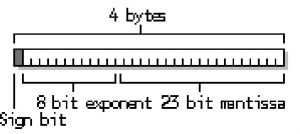Sunday, September 24, 2023 00:37

## Float variable type

C# language uses float variable type to store real number values in floating point (negative and positive numbers that contain a fractional part). The C# compiler will allocate 32 bits (4 bytes) to store values of type float. A float variable type can store values with a precision of six or seven decimals in the interval of 3.4E-38 and 3.4E+38.

The C# language keeps the value on a mantissa of 23 bits (which contains the fractional part) and an exponent of 8 bits (which contains the power with which the number is multiplied) and a single bit of sign (which tells the compiler if the value is positive or negative). In other words, if a variable contains the value 3.4E+38, the sign bit will be 0, which indicates a positive number, the 23 bits mantissa will contain a binary representation of number 3.4, and the 8 bit exponent will contain a binary representation of 1038This section, as many others that will follow, represents real numbers using scientific notation. This system allows to represent any number utilizing a single digit to the left of the point, an unlimited number of digits to the right of the point, and an exponent using a power of 10. When you calculate the real value of the number, multiply the number (mantissa) with the value of 10 to the power (where x represents the exponent). For instance, number 3.1415967E+7 equals to 31415967.0, or 3.1415967 * 107.

If you want a numeric real literal to be treated as float, use the suffix f or F, for example:

Without the suffix f, the number is treated as a double and generates a compiler error.

The concepts explained in this lesson are also shown visually as part of the following video:

EXERCISES
1. Write an expression that calculates the area of a trapezoid by given sides a, b and height h.

Solution

Guidelines: The formula for trapezoid surface is: S = (a + b) * h / 2.